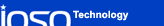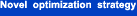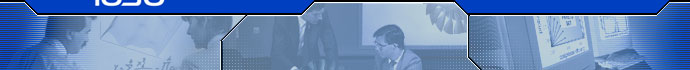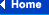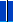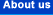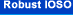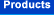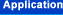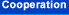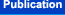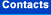### Typical examples of optimization problems

 Problem The purpose and constraints Type of object representation Number of variables/ constraints The class  of goal function Number of solved problems Optimization of geometrical parameters of the multistage axial compressor 1. The magnification of efficiency on design operational mode of gas-turbine engine or power installation at observance of constraints on air consumption, compressor operation stability and strength of details. Mathematical model 4 - 99/ 8 - 220 Multiextreme, nondifferentiable > 60 2. The magnification of compressor efficiency on several operational modes of gas-turbine engine at observance of constraints on air consumption, operation stability and strength of details. (Multicriteria approach). Mathematical model 4 - 99/ 8 - 600 Multiextreme, nondifferentiable > 40 3. The maximization of efficiency ensured with preset probability at a preset accuracy of details manufacturing and preset probability of constraints insurance. Mathematical model 4 - 63/ 12 - 280 Stochastic > 30 The joint optimization of geometrical parameters and blades rows control laws of multistage axial compressor The maximization of efficiency for a specific range of operational modes at observance of constraints on air consumption, compressor operation stability and strength of details Mathematical model 4-63/ 8 - 250 Multiextreme, nondifferentiable 12 The optimization of work process parameters of gas-turbine engine of versatile aircraft The choice of optimal values of design and gas-dynamic parameters of gas-turbine engine for given totality of aircraft flight modes Mathematical model 3/4 Nonconvex 2 The searching of Pareto-optimal set of multistage axial compressor parameters and control laws for a preset set of engine operational modes. The minimization of fuel consumption for each of specified operational modes at observance of restrictions on operation stability and strength of details. (Multicriteria optimization). Mathematical model 6 - 18/6 Multiextreme 3 The optimization of control law of the serial gas-turbine engine controllable elements. Searching of positions of controllable elements to be Pareto-optimal on "thrust" and "fuel consumption" criteria at observance of constraints on components stability margins and on gas temperature at turbine inlet. (Multicriteria optimization). Mathematical model 3 - 4/5 Nonconvex 10 The multipurpose optimization of STOVL aircraft power plant design parameters and control laws. The magnification of vertical thrust and low pressure compressor stability margins at observance of constraints on other elements stability margins, temperatures of gas at turbine inlet and nozzles outlets, on rotors rotation rates and on the thrust modulation margin Mathematical model 8/6 Nonconvex 9 The multipurpose optimization of time control laws of power plant of STOVL aircraft for it’s short take-off The decreasing of take-off run length and fuel consumption for STOVL aircraft short take-off from it’s start to evolutive velocity and safe altitude reaching at observance of constraints on stability margins, temperature of gas and rotors rotation rates Mathematical model Up to 53/8 Multiextreme 6 The optimization of control laws of   aircraft gas-turbine engine at non-stationary perturbations of inlet stream 1. The preservation of steady engine operation at minimum losses of thrust impulse for specified parameters of perturbation. Mathematical model 48-216/ 26 Multiextreme > 20 2. The maximization of probability of steady operation and minimization of expectation of thrust impulse losses for specified perturbation probability performances. Mathematical model 24-112/ 26 Stochastic 13 3. Raise of probability of steady operation at a lack of information about perturbation parameters. Mathematical model 16-48/5 Multiextreme 8 The optimization of aircraft gas-turbine engine controllable elements control laws at maneuvering of aircraft. The minimization of maneuver execution time and fuel consumption at constraint on aircraft overload and admissible angles of attack and on engine operation stability. Mathematical model 20 - 70/5 Multiextreme > 20 The optimization of parameters and control programs of gas-turbine engines, which have completed resource, in a structure of ground power installations The minimization of fuel consumption, the minimization of thrust and maximization of outlet temperature for gas-turbine engine in a structure of the thermal machine. (Multicriteria approach) Mathematical model + actual plant 3 - 5/3 Multiextreme 3 The optimal calibration of the microprocessor control system of automobile engine. The minimization of fuel consumption at specified constraints on exhaust toxicity Actual plant on the experimental bench 3/3 Stochastic 2 The optimization of multilayer optical coatings. Minimization of surface reflection (absorption) coefficient in a specified range of lengths of waves Mathematical model 6 - 20/57 Multiextreme, ravine 6